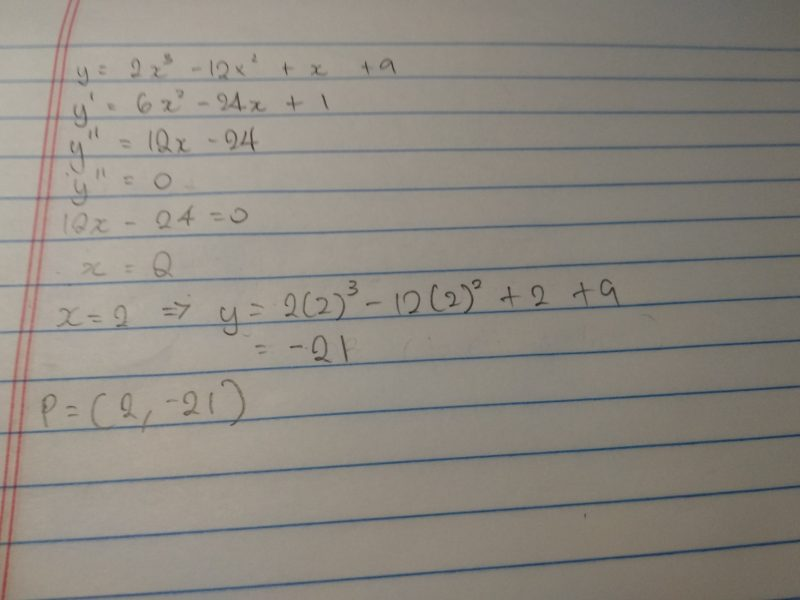# Question

Point P moves along the curve y=2X^3 -12X^2 +X +9. Find the coordinates of P such that the normal to the curve at P will have a gradient that is either a maximum or minimum.

Please help with this question. TIA

1 Answer

# Answer1 Reply 0 Likes

Given answer is also the same. I do not understand.

I thought y’ should be zero for the gradient to be max or min.

and y” should be less than 0(for max) and greater than 0(for min).

I understand that if the gradient of tangent is max then the gradient of normal is min. So we can find the gradient of tangent cos it is either max or min.

If possible please explain why y” should be zero and not y’

Thank you

0 Replies 0 Likes